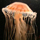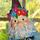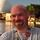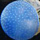## General Question# Matrices to solve a vector combination problem.

Asked by dotlin (422) August 10th, 2010

So I’m just starting out learning this at home.

Lets say we have two vectors

a=[3/-6] <6 below the 3 b=[2/6] again the 6 is below.

a+b=c =[7/6]

Then the book goes off into saying

ax+by=c but in matrix form, there’s a graph of a, b and c next to it and I know x in ax is suppose to be the x coordinates and y is the y coordinates, but what x and y coordinates?

[3/-6]x+[2/6]y=[7/6]

[3/-6]x+[2/6]y= [3, 2/-6, 6][x/y]

the book says 3 x X = 7 but when did we work out what x was and the same with y

Observing members: 0Composing members: 0I am completely confused by your notation…

lilikoi (10084)“Great Answer” (0) Flag as…Your very first equation doesn’t make sense. In Matrix Addition, the sum matrix is comprised of the sums of the corresponding elements of the original matrices.

`|3| |2| |5|`
`| |+| |=| |`
`|-6||6| |0|`

That is, the matrix of 3 over -6 plus the matrix of 2 over 6 equals the matrix of 5 over 0, not 7 over 6.

MrItty (17381)“Great Answer” (1) Flag as…X=1
Y=2

SolsticeRG (76)“Great Answer” (0) Flag as…That is, assuming you’ve simply represented linear simultaneous equations:

3X + 2Y = 7
-6X + 6Y = 6,

which can we rewritten in matrix form:

| 3 2 | |X| = |7|
|-6 6 | |Y| = |6|, where this is basically in the form: [A][B] = [C].

Rewriting, you can use inv[A][C] = [B], which solves the linear system using inverse matrices.

A much easier way to do this is through simple elimination (or even substitution). For instructions on that, please see 7th Grade.

SolsticeRG (76)“Great Answer” (0) Flag as…or